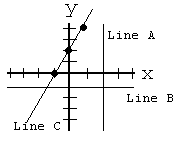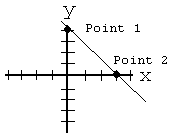# The Equation of a Line

 Our Algebra 1 tutorial software program contains over 60 topic areas. One of them is Equation of a Line, and this page summarizes the main ideas of this topic. This page is intended for review, and is not a substitute for the interactive, self-paced tutorials of the MathTutor algebra 1 software program.

General Explanation

Three different types of equations can be used to describe a straight line. The most commonly used form is called Slope-Intercept Form, and it is the form we will use in this problem type. Using the slope-intercept form, the equation of a straight line looks like this:

`y = mx + b`
where m is the slope of the line, and b is the y-intercept. Let's define those terms.

The slope of a line is a number which indicates how steep the line is. The slope of a line can be calculated if you know the coordinates of two points on the line. Let's assume point 1 on the line has coordinates (x1,y1) and point 2 on the line has coordinates (x2,y2). Then we can calculate the slope of the line using this formula:

`Slope = (y2 - y1) / (x2 - x1)`
Another common way of calculating the slope of a line is this:
`Slope = m = rise / run`
where "rise" is the amount the y-coordinate changes in going from one point to another, and "run" is the amount the x-coordinate changes in going between the same points. You should be able to see that the first and second ways shown above of calculating slope are really the same, because (y2 - y1) shows how much y changes in going between two points (which is the same as "rise") and (y2 - y1) shows how much x changes in going between two points (which is the same as "run").

To determine the equation of a straight line, follow these steps:

1. Determine the y-intercept by looking at the graph and seeing what the value of y is at the point where the line crosses the y-axis. This number will be b in the slope-intercept form equation.
2. Determine the slope of the line. To do this pick any two points on the line, determine the coordinates of each points, and do the calculation shown above to find the slope.
3. Plug in the values you found for the y-intercept and the slope in in the standard equation for a straight line:
`y = mx + b`
For instance, if you find that the y-intercept is 3 and the slope is 0.5, then the equation of the straight line is:
`y = 0.5x + b`
If the line is vertical or horizontal, then the equation of the line will be different than y = 0.5x + b.
• If the line is horizontal, then the value of y is the same at all points along the line, while x can vary. The equation of a horizontal line is always of the form:
`y = C`
where C is the value of y at the point where the line crosses the y-axis.
• If the line is vertical, then the value of x is the same at all points along the line, while y can vary. The equation of a vertical line is always of the form:
`x = C`
where C is the value of x at the point where the line crosses the x-axis.
Consider the figure below for examples of straight lines.
``
Line A is a vertical line and crosses the x-axis at x = 2. Therefore, the equation of line A is: x = 2.

Line B is a horizontal line and crosses the y-axis at y = -1. Therefore, the equation of the line is: y = -1.

Line C will have an equation of the form y = mx + b. Since it crosses the vertical axis at (0,2), b is equal to 2. The slope of the line can be found using the formula Slope = (y2 - y1) / (x2 - x1) for any two points on the line. Consider the points (1,4) and (-1,0). Plugging these points into the formula we get m = (4 - 0) / (1 - (-1)) = 4/2 = 2. So the equation of line C is y = 2x + 2.

Sample Problem

Find the equation of the line in the figure below:
``

Solution

Since the line is neither horizontal nor vertical, its equation will be in the form y = mx + b.

We can see, by inspection, that the line crosses the vertical axis at the point (0,4). So, b = 4.

To find the slope, we need to know the coordinates of any two points on the line. let's use the point where the line crosses the x-axis, and the point where the line crosses the y-axis, or (3,0) and (0,4). So, to calculate slope:

`Slope = m = (y2 - y1) / (x2 - x1) = (0 - 4)/(3 - 0) = -4/3`
So, the equation of the line is:
`y = (-4/3)x + 4`